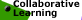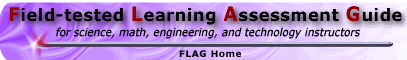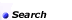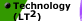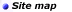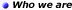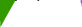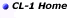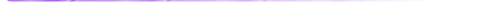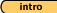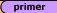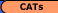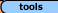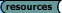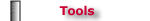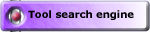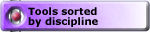## Tools - Math 'Creating Measures' Square-ness Task, Example #1 (solution)

Square-ness, Example #1 (solution) || Steep-ness, Example #2 (solution)
Compact-ness, Example #3 (solution) || Crowded-ness, Example #4 (solution)
Awkward-ness, Example #5 (solution) || Sharp-ness, Example #6 (solution)

Malcolm Swan
Mathematics Education
University of Nottingham
Malcolm.Swan@nottingham.ac.uk

Jim Ridgway
School of Education
University of Durham
Jim.Ridgway@durham.ac.uk

 This problem gives you the chance to: criticise a given measure for the concept of "square-ness" invent your own ways of measuring this concept examine the advantages and disadvantages of different methods.Warm-up

Use visual judgements to answer the warm-up questions.
Which rectangle looks the most square?
Which rectangle looks least square?

Without measuring anything, put the rectangles in order of "square-ness."

Comment:
This first question is simply intended to orientate the students to the task in hand. It may be used as a class discussion.

1. Someone has suggested that a good measure of "square-ness" is to calculate the difference:

Longest side - shortest side

for each rectangle. Use this definition to put the rectangles in order of "square-ness." Show all your work.

Solution:
Using the measure 'Longest side - shortest side', the "square-ness" of each rectangle is given in the table below (using centimeters as the unit).

 Rectangle A B C D E F G H I Dimensions (cm) 3 x 3 1 x 8 6 x 2 4 x 1 3 x 4 3 x 2 6 x 5 4 x 2 12 x 4 Square-ness (cm) 0 7 4 3 1 1 1 2 8

Using this measure, the rectangles in order from most to least square are:

A, E and F and G (tie), H, D, C, B, I.

1. Using your results, give one good reason why Longest side - shortest side is not a suitable measure for "square-ness."

Solution:
The above measure is unsatisfactory because:

• It gives no indication of the overall 'proportions'. (E, F and G under this definition have the same square-ness yet are clearly different in shape, while C and I are similar in shape but give different square-ness measures).

• It is dependent on the units used. If we use inches instead of centimetres we get a different "square-ness" measure.

1. Invent a different way of measuring "square-ness." Describe your method carefully below:

Solution:
There are many other ways of measuring "square-ness." Students might, for example, propose using:

1. The ratio longest side/shortest side;

2. The largest angle between the diagonals of the rectangle;

3. The ratio of perimeter/area.
a) and b) seem equally sensible. c), however, suffers the same problem as before. As it is not dimensionless, an enlargement of a rectangle will result in a different value for its "square-ness."

If, however, we use

1. the ratio (perimeter)2 / area

then we would have a suitable, dimensionless measure.

1. Place the rectangles in order of "square-ness" using your method. Show all your work.

Solution:
Whichever measure we now use (a), (b) or (d), we obtain the same order for the rectangles. In order of "square-ness" they are:

A (most square), G, E, F, H, C and I (tie), D, B (least square).
 Rectangle A B C D E F G H I Dimensions (cm) 3 x 3 1 x 8 6 x 2 4 x 1 3 x 4 3 x 2 6 x 5 4 x 2 12 x 4 Ratio:longest ÷ shortest 1 8 3 4 1.3 1.5 1.2 2 3 Largest angle between diagonals 90o 166o 143o 152o 106o 113o 100o 127o 143o Ratio: Perimeter2 ÷ area 16 40.5 21.3 25 16.3 16.7 16.1 18 21.3

1. Do you think your measure is a good way of measuring "square-ness?" Explain your reasoning carefully.

Solution:
Here we would like students to review their results critically and decide whether the results from their measurements accord with their intuitions.

1. Find a different way of measuring "square-ness."
Compare the two methods you invented. Which is best? Why?

Solution:
This question provides an opportunity for students to look for an alternative measure.

Square-ness, Example #1 (solution) || Steep-ness, Example #2 (solution)
Compact-ness, Example #3 (solution) || Crowded-ness, Example #4 (solution)
Awkward-ness, Example #5 (solution) || Sharp-ness, Example #6 (solution)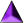Introduction || Assessment Primer || Matching Goals to CATs || CATs || Tools || Resources

Search || Who We Are || Site Map || Meet the CL-1 Team || WebMaster || Copyright || Download
College Level One (CL-1) Home || Collaborative Learning || FLAG || Learning Through Technology || NISE